The FD-VBPM based on the E and H fields are equivalent and yield almost identical results .
Similar to the vector wave equation for the electric field, the equation for the magnetic field considering transversely scaled version of PML is: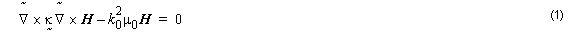here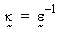.

The double-curl Equation 1 involves three vector components of the magnetic field, while strictly only two are needed.

Incorporating the divergence-free condition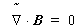into Equation 1, we can reduce the number of components in the field equation to the two transverse components of the magnetic field hx and hy only. To achieve this purpose, we separate the transverse and longitudinal components of Equation 1.

On the transverse plane, Equation 1 becomes: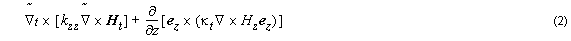Now, using an appropriate reference refractive index n0 , and slowly varying envelope
approximation (SVEA), we assume the following form of the solution: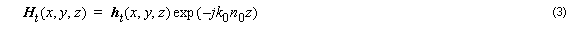By using Equation 3 into Equation 2, we can recast Equation 1 in the following form: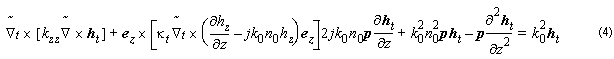with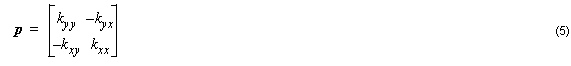From divergence condition, we get: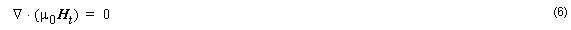By using Equation 3 into Equation 6, we get: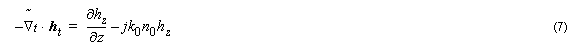By substituting Equation 7 into Equation 4, we get the following vectorial wave equation written in terms of the transverse components of magnetic field: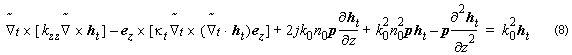or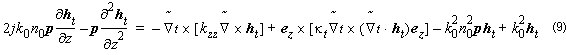To obtain Equation 9, we assume that the permittivity along the propagation direction
is often slow, as is observed in real devices. Therefore, ∂ p ⁄ ∂z has been neglected.

Similar to the E-formulation, the elimination of the axial component using the
divergence condition.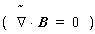guarantees the complete elimination of spurious modes, and drastically reduced computation efforts and resources, compared to the formulation which uses three field components.

We can rewrite Equation 9: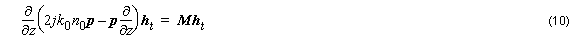where the operator Mht  is defined as: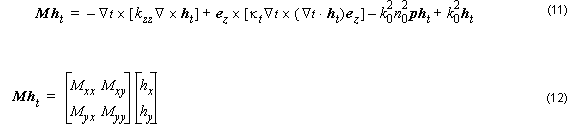Here, the matrix M can also be written in components: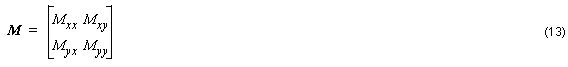The differential operators are defined by the following equations: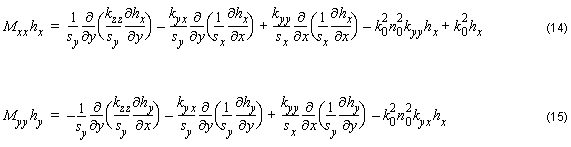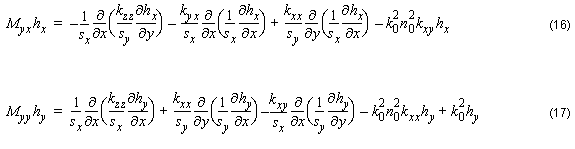The discontinuities of ∂hy ⁄ ∂x and ∂hx ⁄ ∂y across the index interfaces along y and x directions are responsible for the polarization dependence ( i. e. hxx hyy ) and coupling ( i. e. hxy  and hyx ≠ 0 ).

The solution to Equation 9 can be written in a exponential form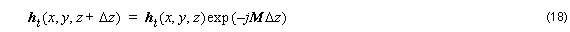which can also be approximated by a weighed finite-difference form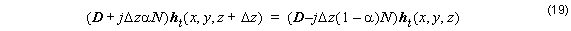Here we get D = p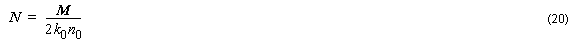for paraxial approximation and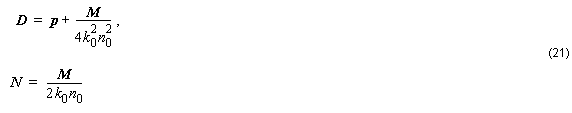As in the E-Formulation description, we can apply the Padé recursion formula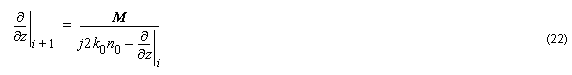AS in E-formulation, we can derive wide-angle BPM considering high order Padé, as the parameter a is introduced to control the schemes used to solve the finite- difference equations.

We can recast Equation 19 in the following form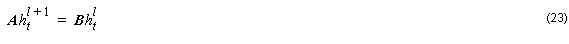where hlt  and ht + 1  are field vectors at two sequential steps l and l + 1 , A and B are nonsymmetric complex band matrices. By solving Equation 23, we can simulate the propagation of the beam in anisotropic materials, such as the polarization dependence and coupling, due to both the material and geometrical effects.

The system in Equation 23 is solved efficiently by the well established sparse matrix solver “bicgstab” (BiConjugate Gradients Stabilized method ).